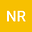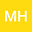About a fixed-point-type transformation to solve quadratic matrix equations using the Krasnoselskij method.
••Miguel Hernandez
Author Profile## Abstract

In this paper, we study the simplest quadratic matrix equation: $\mathcal{Q}(X)=X^2+BX+C=0$. We transform this equation into an equivalent fixed-point equation and based on it we construct the Krasnoselskij method. From this transformation, we can obtain iterative schemes more accurate than successive approximation method. Moreover, under suitable conditions, we establish different results for the existence and localization of a solution for this equation with the Krasnoselskij method. Finally, we see numerically that the predictor-corrector iterative scheme with the Krasnoselskij method as a predictor and the Newton method as corrector method, can improves the numerical application of the Newton method when approximating a solution of the quadratic matrix equation.
27 Sep 2021Submitted to Mathematical Methods in the Applied Sciences
28 Sep 2021Submission Checks Completed
28 Sep 2021Assigned to Editor
02 Oct 2021Reviewer(s) Assigned
21 Nov 2021Review(s) Completed, Editorial Evaluation Pending
30 Nov 2021Editorial Decision: Revise Minor